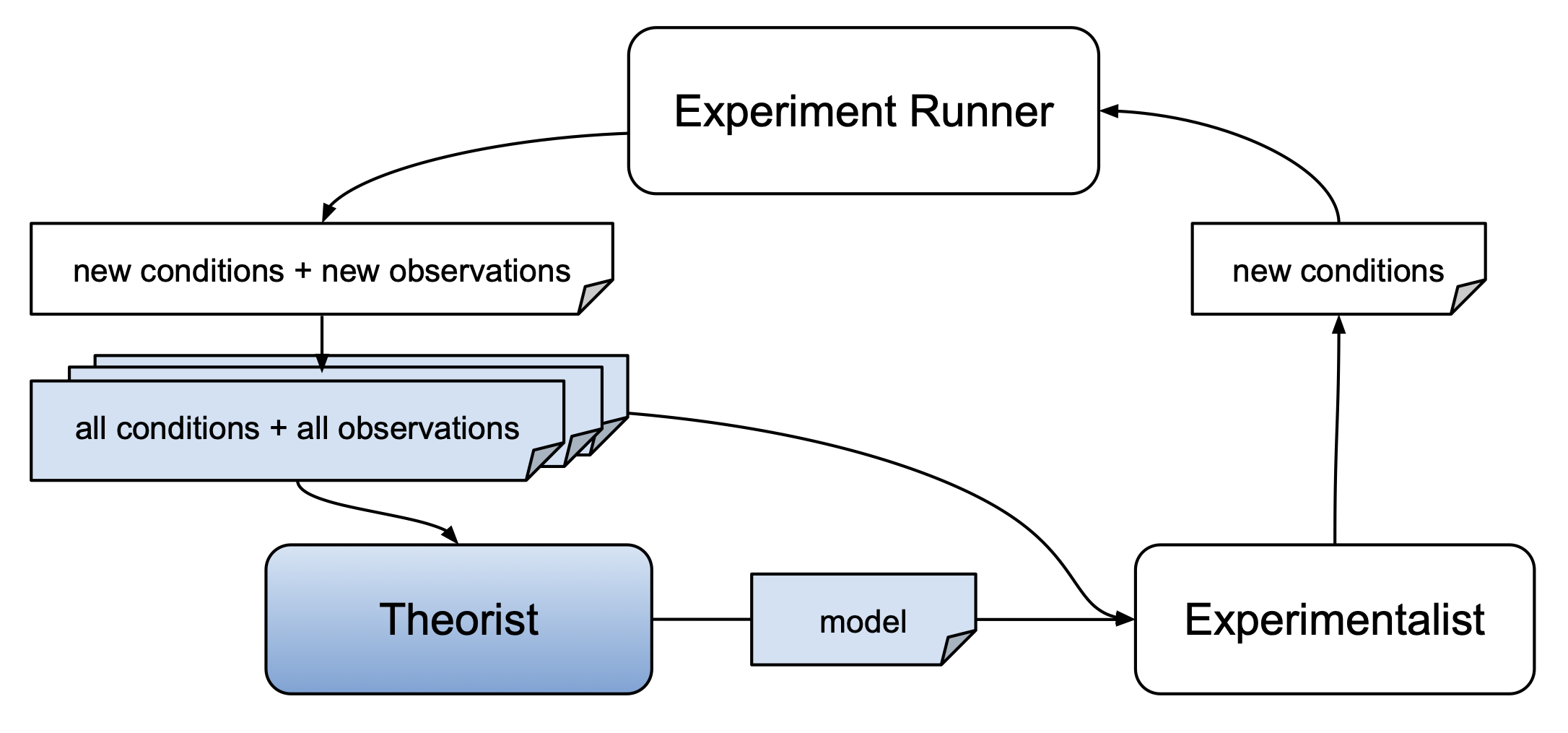# Contribute A Theorist

AutoRA theorists are meant to return scientific models describing the relationship between experimental conditions and observations. Such models may take the form of a simple linear regression, non-linear equations, causal graphs, a more complex neural network, or other models which - can be identified based on data (and prior knowledge) - can be used to make novel predictions about observations given experimental conditions.All theorists are implemented as sklearn regressors. They are fitted based on experimental conditions and respective observations, and can be used to predict observations for new experimental conditions.

## Repository Setup

We recommend using the cookiecutter template to set up a repository for your theorist. Alternatively, you use the unguided template. If you are using the cookiecutter template, you can set up your repository using

cookiecutter https://github.com/AutoResearch/autora-template-cookiecutter


Make sure to select the theorist option when prompted. You can skip all other prompts pertaining to other modules (e.g., experimentalists) by pressing enter.

## Implementation

Once you've created your repository, you can implement your theorist by editing the init.py file in src/autora/theorist/name_of_your_theorist/. You may also add additional files to this directory if needed. It is important that the init.py file contains a class called NameOfYourTheorist which inherits from
sklearn.base.BaseEstimator and implements the following methods:

• fit(self, conditions, observations)
• predict(self, conditions)

See the sklearn documentation for more information on how to implement the methods. The following example init.py illustrates the implementation of a simple theorist that fits a polynomial function to the data:

"""
Example Theorist
"""

import numpy as np
from sklearn.base import BaseEstimator

class ExampleRegressor(BaseEstimator):
"""
This theorist fits a polynomial function to the data.
"""

def __init__(self, degree: int = 2):
self.degree = degree

def fit(self, conditions, observations):

# polyfit expects a 1D array
if conditions.ndim > 1:
conditions = conditions.flatten()

if observations.ndim > 1:
observations = observations.flatten()

# fit polynomial
self.coeff = np.polyfit(conditions, observations, 2)
self.polynomial = np.poly1d(self.coeff)
pass

def predict(self, conditions):
return self.polynomial(conditions)